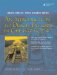# Structs

In the C language, there is the struct keyword, for defining a structured chunk of memory.

Example 2.1. src/structdemo/demostruct.h

 ```[ . . . . ] struct Fraction { int numer, denom; string description; }; [ . . . . ] ```

A structured piece of memory is comprised of smaller chunks of memory of various types and sizes, each chunk accessible by name. Example 2.1 shows the definition of a struct.

Example 2.2 shows how we can use a structured chunk of memory (containing subobjects) as a single entity. Each data member (numer, denom, description) is accessible by name.

Example 2.2. src/structdemo/demostruct.cpp

 ```[ . . . . ] void printFraction (Fraction f) { <-- 1 cout << f.numer << "/" << f.denom << endl; cout << " =? " << f.description << endl; } int main() { Fraction f1; fl.numer = 4; fl.denom = 5; fl.description = "four fifths"; Fraction f2 = {2, 3, "two thirds"}; <-- 2 f1.numer = f1.numer + 2; printFraction (f1); printFraction (f2); return 0; } Output: 6/5 =? four fifths 2/3 =? two thirds ```   (1)Passing a struct by value could be expensive if it has large components. (2)member initializationAn Introduction to Design Patterns in C++ with Qt 4
ISBN: 0131879057
EAN: 2147483647
Year: 2004
Pages: 268